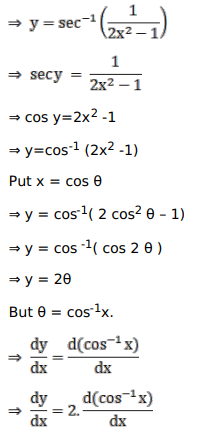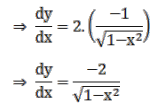# Solve this following

Question:

Mark $(\sqrt{ })$ against the correct answer in the following:

If $y=\sec ^{-1}\left(\frac{1}{2 x^{2}-1}\right)$ then $\frac{d y}{d x}=?$

A. $\frac{-2}{\left(1+x^{2}\right)}$

B. $\frac{-2}{\left(1-x^{2}\right)}$

C. $\frac{-2}{\sqrt{1+x^{2}}}$

D. none of these

Solution: